Searching...

# Tech Tips

The crest factor is the ratio of wave height, which is one of the quality parameters in Alternating Current (AC) power sources and Bipolar power supplies.

In the field of electronics, it is used to describe the state of the AC power source supplied from an outlet. The crest factor is expressed as the ratio of the peak value to the rms value of the alternating current (AC) waveform.

The equation can be expressed as follows.

When the peak value of each waveform is set to 1
Waveform Effective value
(RMS value)
Mean value Waveform factor Crest factor
DC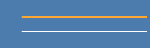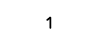Sine wave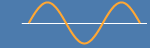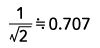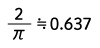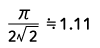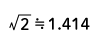Full wave rectified sine wave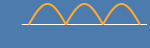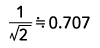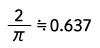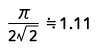Half-wave rectified sine wave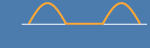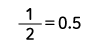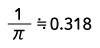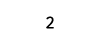Triangle wave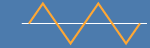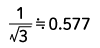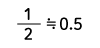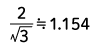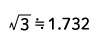Sawtooth wave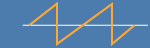Square wave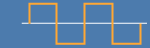PWM signal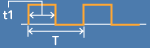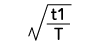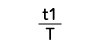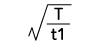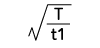Waveform Factor = RMS value / average value
Crest Factor = Peak value / RMS value

Usually, we don't care about the current value when plugging an electric appliance into an outlet. And we are even less conscious of the crest factor. So why should we consider the crest factor?

A high crest factor is called a "poor power factor" and can cause the following problems.

• Heat generation and burnout of power receiving equipment
• Malfunction of electronic equipment
• Input current increases due to higher apparent power

As you can see from the above equation, the higher the peak value of the numerator, the higher the value of the crest factor. A waveform with a high peak value occurs when the current flows in a sharp and pointed manner as shown in the figure below.
This is mainly seen when a circuit method known as a capacitor-input type is used.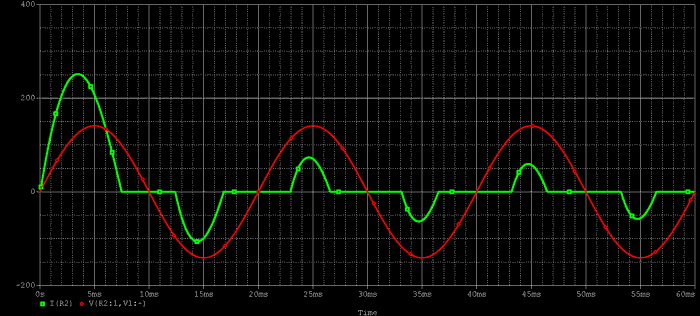A sharp current causes a large current to flow through the capacitor. Capacitors with repeated large currents can make a roaring noise, generate heat, and sometimes burn out. This is the first problem.

Extreme peak current waveforms also contain high-frequency components.
Some electronic devices can react to high-frequency components and cause malfunctions.

Third, as can be seen from the equation, a higher crest factor results in a higher peak current, so the wiring must be correspondingly thicker. If the current = power/voltage is calculated without considering the crest factor, the current capacity of the wiring will be insufficient, and the cause of heat generation and the impedance of the wiring will lead to distortion of the voltage waveform.

To prevent these drawbacks, a power factor correction circuit (PFC) is used to reduce the peak current by bringing the input current closer to a sine wave.
However, PFC is also efficient, so the choice has advantages and disadvantages. If you consult with our sales staff, including your operating environment and usage, we will propose a power supply that matches your needs.